# 齿轮的齿数和基圆

1726 2022-08-10 15:05

==================================我是美丽的分割线----------------------------------------------

``````"m" = 2mm'模数
"z"= 30'齿数
"alpha"= 20'压力角
"D"= "m" * "z"'分度圆直径
"Db"= "D" * cos ( "alpha" )'基圆直径
"Df"= "m" * ( "z" - 2.5 )'齿根圆直径
"Da"= "m" * ( "z" + 2 )'齿顶圆直径
"P"= pi * "m"'齿距
"s"= "P" / 2'齿厚
"r"= 0.38 * "m"'齿根过渡圆弧半径
"ha*"= 1'齿顶高系数
"c*"= 0.25'顶隙系数
"ha"= "m" * "ha*"'齿顶高
"Df_1"= "m" * ( "z" - 2 * "ha*" - 2 * "c*" )'齿根圆直径_1
"Da_1"= "m" * ( "z" + 2 * "ha*" )'齿顶圆直径_1
"hf"= "m" * ( "ha*" + "c*" )'齿根高

51*cos(pi/9)*(t*sin(t)+cos(t))

51*cos(pi/9)*(sin(t)-t*cos(t))``````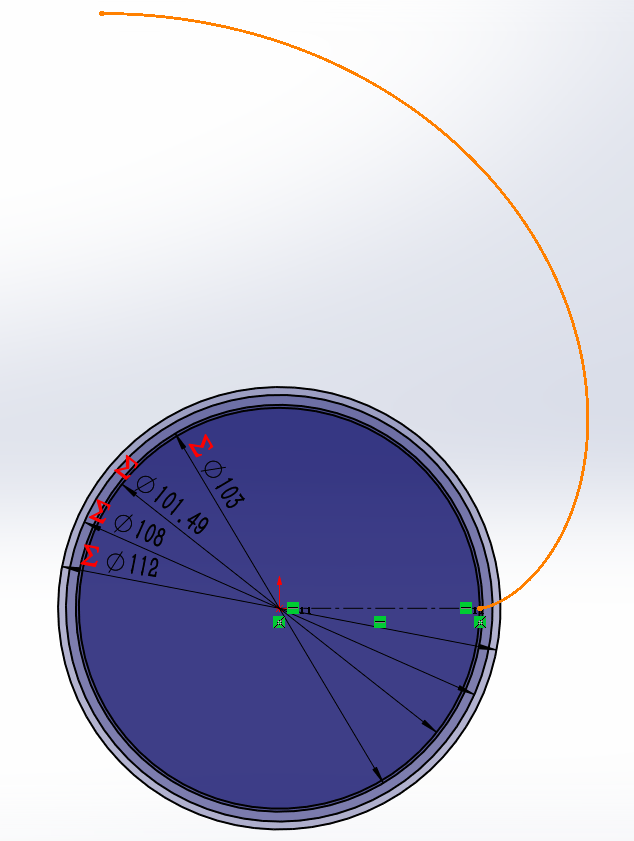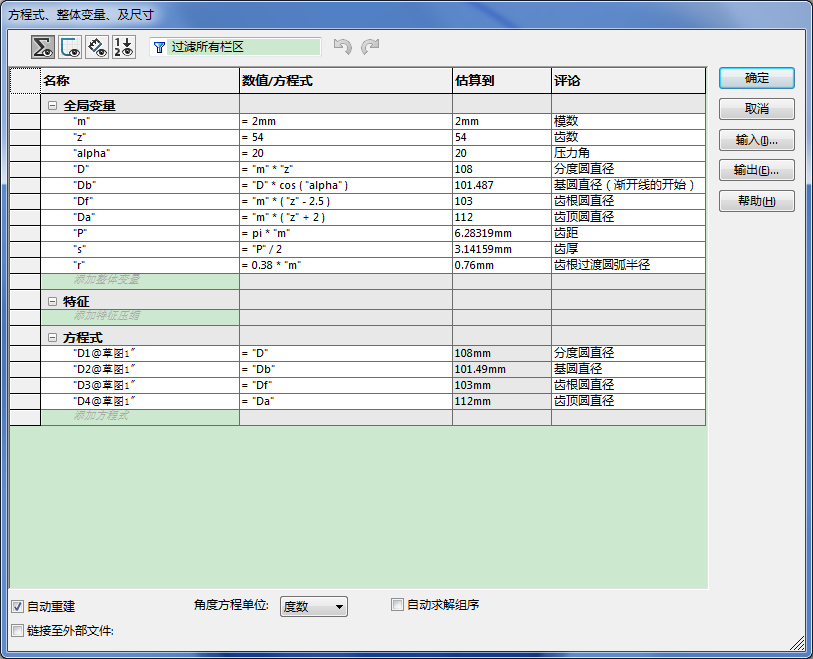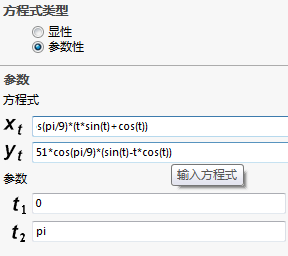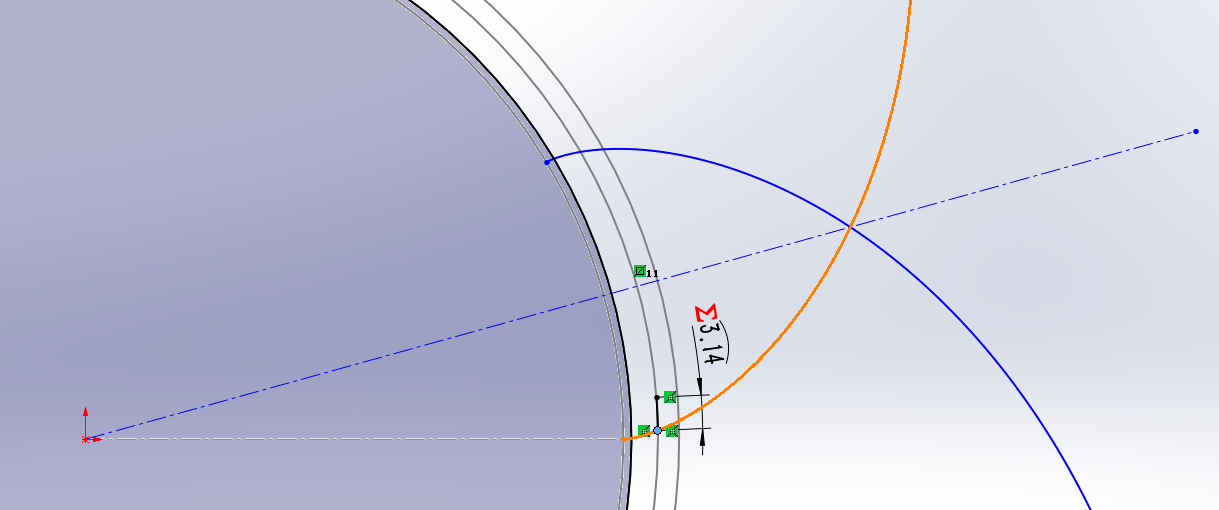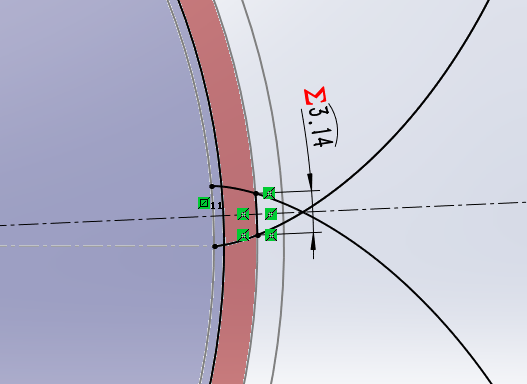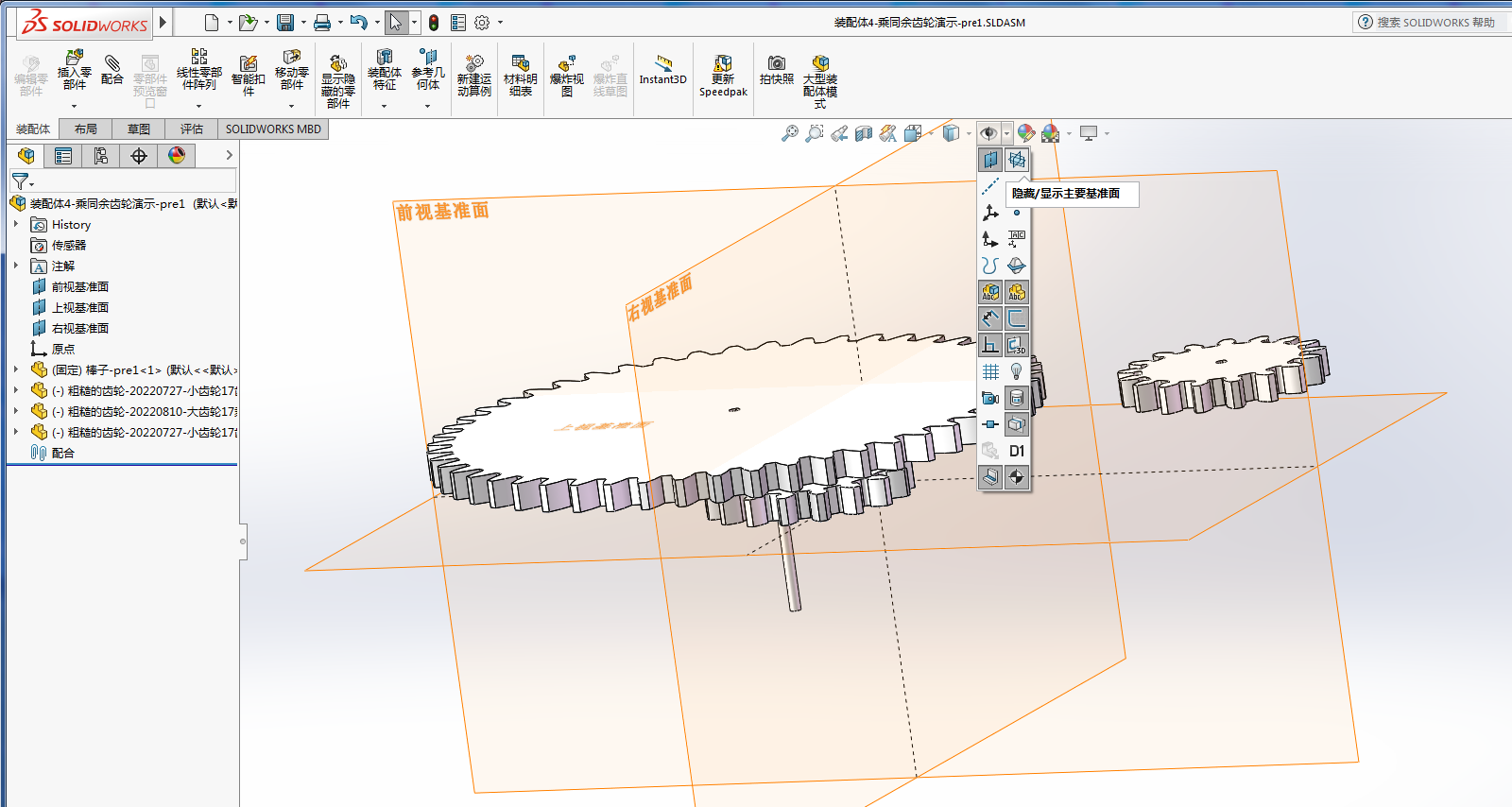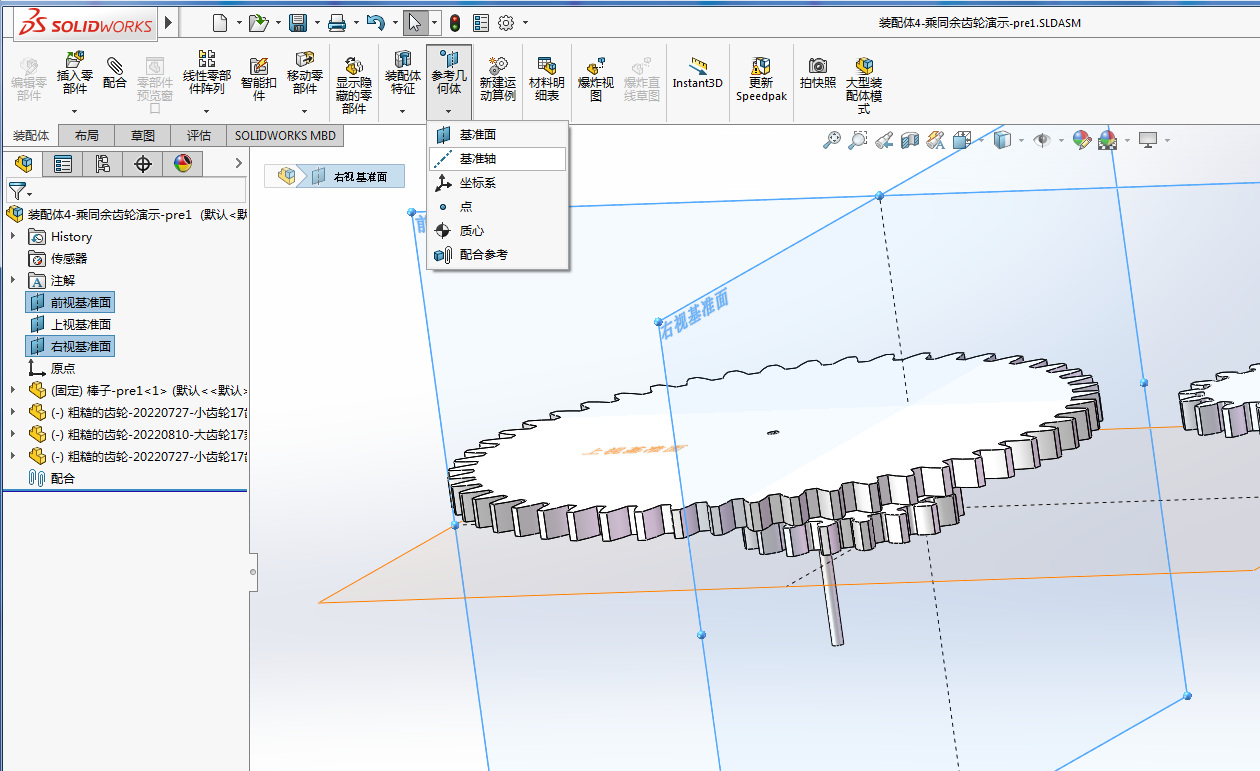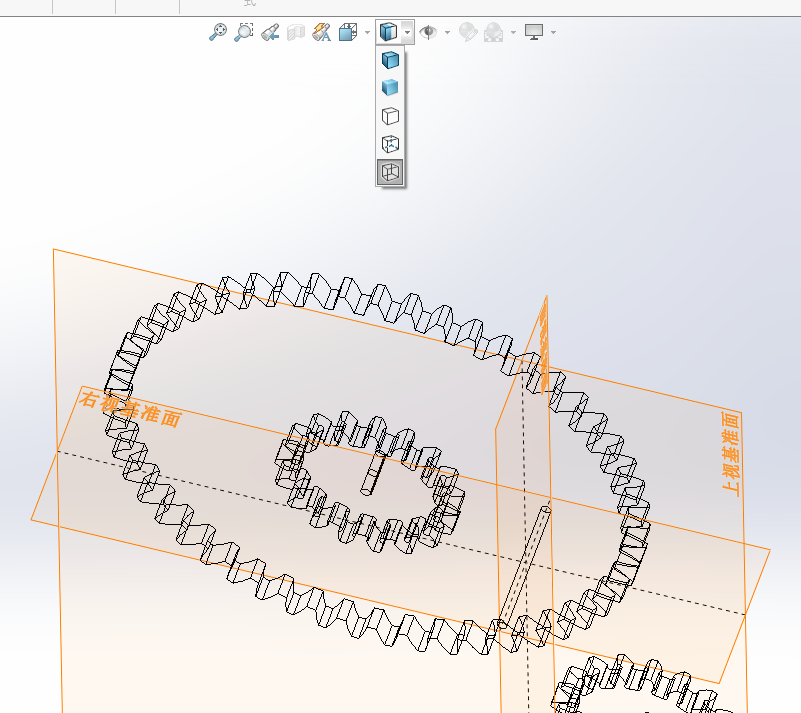·